Home    |    Teacher    |    Parents    |    Glossary    |    About UsLearning algebra is a little like learning another language. In fact, algebra is a simple language, used to create mathematical models of real-world situations and to handle problems that we can't solve using just arithmetic. Rather than using words, algebra uses symbols to make statements about things. In algebra, we often use letters to represent numbers.

Since algebra uses the same symbols as arithmetic for adding, subtracting, multiplying and dividing, you're already familiar with the basic vocabulary.

In this lesson, you'll learn some important new vocabulary words, and you'll see how to translate from plain English to the "language" of algebra.

The first step in learning to "speak algebra" is learning the definitions of the most commonly used words.

Algebraic Expressions | Variables | Coefficients | Constants | Real Numbers | Rational Numbers | Irrational Numbers | Translating Words into Expressions

Algebraic Expressions
An algebraic expression is one or more algebraic terms in a phrase. It can include variables, constants, and operating symbols, such as plus and minus signs. It's only a phrase, not the whole sentence, so it doesn't include an equal sign.

Algebraic expression:

3x2 + 2y + 7xy + 5

In an algebraic expression, terms are the elements separated by the plus or minus signs. This example has four terms, 3x2, 2y, 7xy, and 5. Terms may consist of variables and coefficients, or constants.

Variables
In algebraic expressions, letters represent variables. These letters are actually numbers in disguise. In this expression, the variables are x and y. We call these letters "variables" because the numbers they represent can vary—that is, we can substitute one or more numbers for the letters in the expression.

Coefficients
Coefficients are the number part of the terms with variables. In 3x2 + 2y + 7xy + 5, the coefficient of the first term is 3. The coefficient of the second term is 2, and the coefficient of the third term is 7.

If a term consists of only variables, its coefficient is 1.

Constants
Constants are the terms in the algebraic expression that contain only numbers. That is, they're the terms without variables. We call them constants because their value never changes, since there are no variables in the term that can change its value. In the expression 7x2 + 3xy + 8 the constant term is "8."

Real Numbers
In algebra, we work with the set of real numbers, which we can model using a number line.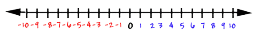Real numbers describe real-world quantities such as amounts, distances, age, temperature, and so on. A real number can be an integer, a fraction, or a decimal. They can also be either rational or irrational. Numbers that are not "real" are called imaginary. Imaginary numbers are used by mathematicians to describe numbers that cannot be found on the number line. They are a more complex subject than we will work with here.

Rational Numbers
We call the set of real integers and fractions "rational numbers." Rational comes from the word "ratio" because a rational number can always be written as the ratio, or quotient, of two integers.

Examples of rational numbers
The fraction ½ is the ratio of 1 to 2.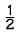Since three can be expressed as three over one, or the ratio of 3 to one, it is also a rational number.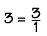The number "0.57" is also a rational number, as it can be written as a fraction.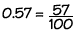Irrational Numbers
Some real numbers can't be expressed as a quotient of two integers. We call these numbers "irrational numbers". The decimal form of an irrational number is a non-repeating and non-terminating decimal number. For example, you are probably familiar with the number called "pi". This irrational number is so important that we give it a name and a special symbol!

Pi cannot be written as a quotient of two integers, and its decimal form goes on forever and never repeats.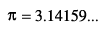Translating Words into Algebra Language
Here are some statements in English. Just below each statement is its translation in algebra.

the sum of three times a number and eight
3x + 8

The words "the sum of" tell us we need a plus sign because we're going to add three times a number to eight. The words "three times" tell us the first term is a number multiplied by three.

In this expression, we don't need a multiplication sign or parenthesis. Phrases like "a number" or "the number" tell us our expression has an unknown quantity, called a variable. In algebra, we use letters to represent variables.

the product of a number and the same number less 3
x(x – 3)

The words "the product of" tell us we're going to multiply a number times the number less 3. In this case, we'll use parentheses to represent the multiplication. The words "less 3" tell us to subtract three from the unknown number.

a number divided by the same number less five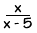The words "divided by" tell us we're going to divide a number by the difference of the number and 5. In this case, we'll use a fraction to represent the division. The words "less 5" tell us we need a minus sign because we're going to subtract five.

 Homework Help | Algebra | The Language of AlgebraEmail this page to a friendSearch·  Definitions·  Order of Operations·  Writing equations·  Writing inequalitiesFirst Glance In Depth Examples WorkoutDefinitions